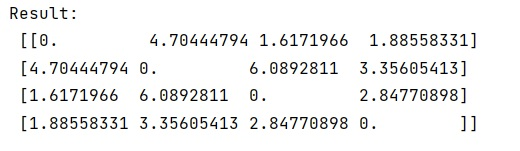# How does condensed distance matrix (cdist) work?

Learn, how does condensed distance matrix work in Python?
Submitted by Pranit Sharma, on January 17, 2023

NumPy is an abbreviated form of Numerical Python. It is used for different types of scientific operations in python. Numpy is a vast library in python which is used for almost every kind of scientific or mathematical operation. It is itself an array which is a collection of various methods and functions for processing the arrays.

## Condensed distance matrix (cdist)

The SciPy library has a spatial module that contains distance.cdist attribute that computes the distance between each pair of the two collections of inputs.

Syntax:

```scipy.spatial.distance.cdist(
XA,
XB,
metric='euclidean',
*,
out=None,
**kwargs
)
```

Parameter(s):

• XA: array_like - A ma by n array of ma original observations in an n-dimensional space. Inputs are converted to float type.
• XB: array_like - An mb by n array of mb original observations in an n-dimensional space. Inputs are converted to float type.
• Metric: str or callable, optional - The distance metric to use. If a string, the distance function can be 'braycurtis', 'canberra', 'chebyshev', 'cityblock', 'correlation', 'cosine', 'dice', 'euclidean', 'hamming', 'jaccard', 'jensenshannon', 'kulczynski1', 'mahalanobis', 'matching', 'minkowski', 'rogerstanimoto', 'russellrao', 'seuclidean', 'sokalmichener', 'sokalsneath', 'sqeuclidean', 'yule'.

Let's understand with the help of an example,

## Python code to demonstrate how does condensed distance matrix (cdist) work?

```# Importing scipy spatial
from scipy.spatial import distance

# Import numpy
import numpy as np

# Creating a data array
arr = [
(35.0456, -85.2672),
(35.1174, -89.9711),
(35.9728, -83.9422),
(36.1667, -86.7833)
]

# Finding distance between each pair of collection
res = distance.cdist(arr,arr, 'euclidean')

# Display result
print("Result:\n",res)
```

Output:What's New (MCQs)

Top Interview Coding Problems/Challenges!

IncludeHelp's Blogs

Languages: » C » C++ » C++ STL » Java » Data Structure » C#.Net » Android » Kotlin » SQL
Web Technologies: » PHP » Python » JavaScript » CSS » Ajax » Node.js » Web programming/HTML
Solved programs: » C » C++ » DS » Java » C#
Aptitude que. & ans.: » C » C++ » Java » DBMS
Interview que. & ans.: » C » Embedded C » Java » SEO » HR
CS Subjects: » CS Basics » O.S. » Networks » DBMS » Embedded Systems » Cloud Computing
» Machine learning » CS Organizations » Linux » DOS
More: » Articles » Puzzles » News/Updates